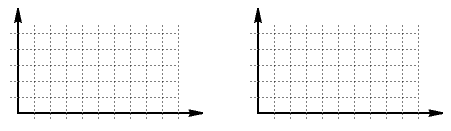### Coulomb's Law Pre-lab Assignment

Print this page, record your answers on it, and show it to your lab TF at the start of your lab session. In this experiment you will investigate the force between charged objects by giving two balls equal charges and placing them at various distances from one another. It is difficult to measure the force one ball exerts on the other, so instead you will measure something that should be proportional to this force.

In the lab the force that one charge exerts on the other is balanced by a force associated with a twisted wire - the force provided by the wire should be proportional to the twist angle of the wire. We'll do something similar here, except that the balancing force will come from an ideal spring.

Click the button to run the simulation. For several positions of the movable charge, record the charge position (r) and the position of the blue rectangle (d, the length of the spring). Then calculate 1/r2 and x, the distance the spring is compressed or stretched compared to its equilibrium length of 10 units.

 Distance between charges (r) Position of blue rectangle (d) 1/r2 x (Note that x = 10 - d) ______ ______ ______ ______ ______ ______ ______ ______ ______ ______ ______ ______ ______ ______ ______ ______ ______ ______ ______ ______ ______ ______ ______ ______

How should the magnitude of the spring force depend on x? Is the force proportional to x, x2, or is there some other relationship?

How do you expect the force that one charge exerts on the other to depend on r?

Plot two graphs, x vs. r and then x vs. 1/r2.Explain why the graphs come out the way they do.